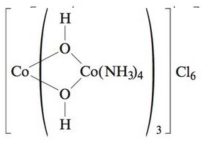# Until the discoveries of Alfred Werner, it was thought that carbon had to be present in a compound for it to be optically active. Werner prepared the following compound containing OH − ions as bridging groups and separated the optical isomers. a . Draw structures of the two optically active isomers of this compound. b. What are the oxidation states of the cobalt ions? c . How many unpaired electrons are present if the complex is the low-spin case?### Chemistry: An Atoms First Approach

2nd Edition
Steven S. Zumdahl + 1 other
Publisher: Cengage Learning
ISBN: 9781305079243

#### Solutions

Chapter
Section### Chemistry: An Atoms First Approach

2nd Edition
Steven S. Zumdahl + 1 other
Publisher: Cengage Learning
ISBN: 9781305079243
Chapter 20, Problem 74AE
Textbook Problem
3 views

## Until the discoveries of Alfred Werner, it was thought that carbon had to be present in a compound for it to be optically active. Werner prepared the following compound containing OH− ions as bridging groups and separated the optical isomers.a. Draw structures of the two optically active isomers of this compound.b. What are the oxidation states of the cobalt ions?c. How many unpaired electrons are present if the complex is the low-spin case?(a)

Interpretation Introduction

Interpretation: The optically active isomers of the given compound are to be drawn. Oxidation state of the cobalt ions and the number of unpaired electrons present if the complex is the low spin are to be stated.

Concept introduction: The electrons in the d orbital of a transition metal split into high and low energy orbitals when ligands are attached to it. The energy difference between these two levels depends upon the properties of both metal and the ligands. If the ligand is strong, then splitting will be high and the complex will be low spin. If the ligand is weak, then splitting will be less and the complex will be high spin.

To determine: The optically active isomers of the given compound.

### Explanation of Solution

The optically active isomers of the given compound are shown in Figure 1.

The optical isomers of the given compound are,

(b)

Interpretation Introduction

Interpretation: The optically active isomers of the given compound are to be drawn. Oxidation state of the cobalt ions and the number of unpaired electrons present if the complex is the low spin are to be stated.

Concept introduction: The electrons in the d orbital of a transition metal split into high and low energy orbitals when ligands are attached to it. The energy difference between these two levels depends upon the properties of both metal and the ligands. If the ligand is strong, then splitting will be high and the complex will be low spin. If the ligand is weak, then splitting will be less and the complex will be high spin.

To determine: The oxidation states of cobalt ions in the compound.

(c)

Interpretation Introduction

Interpretation: The optically active isomers of the given compound are to be drawn. Oxidation state of the cobalt ions and the number of unpaired electrons present if the complex is the low spin are to be stated.

Concept introduction: The electrons in the d orbital of a transition metal split into high and low energy orbitals when ligands are attached to it. The energy difference between these two levels depends upon the properties of both metal and the ligands. If the ligand is strong, then splitting will be high and the complex will be low spin. If the ligand is weak, then splitting will be less and the complex will be high spin.

### Still sussing out bartleby?

Check out a sample textbook solution.

See a sample solution

#### The Solution to Your Study Problems

Bartleby provides explanations to thousands of textbook problems written by our experts, many with advanced degrees!

Get Started

Find more solutions based on key concepts
The water-soluble vitamins are characterized by all of the following except: excesses are stored and easily bui...

Nutrition: Concepts and Controversies - Standalone book (MindTap Course List)

What purpose do the colors in a representational-color image or map serve?

Horizons: Exploring the Universe (MindTap Course List)

What is physically exchanged during crossing over?

Human Heredity: Principles and Issues (MindTap Course List)

47. Name each alkyne:

Chemistry In Focus

Review. Prove that one solution of the equation 2.00x43.00x3+5.00x=70.0 is x = 2.22.

Physics for Scientists and Engineers, Technology Update (No access codes included)